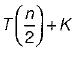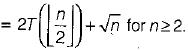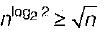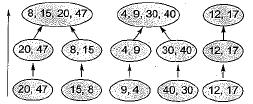Courses

# Divide And Conquer (Basic Level) - 2

## 10 Questions MCQ Test Question Bank for GATE Computer Science Engineering | Divide And Conquer (Basic Level) - 2

Description
This mock test of Divide And Conquer (Basic Level) - 2 for Computer Science Engineering (CSE) helps you for every Computer Science Engineering (CSE) entrance exam. This contains 10 Multiple Choice Questions for Computer Science Engineering (CSE) Divide And Conquer (Basic Level) - 2 (mcq) to study with solutions a complete question bank. The solved questions answers in this Divide And Conquer (Basic Level) - 2 quiz give you a good mix of easy questions and tough questions. Computer Science Engineering (CSE) students definitely take this Divide And Conquer (Basic Level) - 2 exercise for a better result in the exam. You can find other Divide And Conquer (Basic Level) - 2 extra questions, long questions & short questions for Computer Science Engineering (CSE) on EduRev as well by searching above.
QUESTION: 1

### Which of the following is useful in implementing heap sort?

Solution:

For implementation of function call in heap sort, stack is used.

QUESTION: 2

### Following algorithm (s) can be used to sort n integers in the range [1 ... n3] in O(n) time?

Solution:

Radix sort is a non-comparative integer sorting algorithm that sorts data with integer keys by grouping keys which share same position and value. So it take O(n) time.

QUESTION: 3

### The recurrence relation that arises in relation with the complexity of binary search is

Solution:

Binary search only half of the array.
So,QUESTION: 4

Which one of the following statements is false?

Solution:

Connected components of a graph can be computed in linear time by using either breadth- first search or depth-first search.

QUESTION: 5

Merge sort uses

Solution:

A merge sort is comparisbn based sorting algorithm and divide-and-conquer algorithm.

QUESTION: 6

For merging two sorted lists of sizes m and n into a sorted list of size m + n, we required comparisons of

Solution:

The number of comparisons required in the worst case is O(m + n).

QUESTION: 7

Quicksort is run on two inputs shown below to sort in ascending order:
(i) 1 , 2 , 3 .... n .
(ii) n, n - 1, n - 2 , .... 2, 1

Let C1, and C2 be the number of comparisons made for the inputs (i) and (ii) respectively. Then,

Solution:

Both of given cases are Quicksort Worst cases problem, so comparisons are equal.

QUESTION: 8

Let T(n) be the function defined by T(1) = 1, T(n)Which of the following statements is true?

Solution:

Use Master TheoremQUESTION: 9

A sorting technique is called stable if

Solution:

A sorting algorithm is called stable if it keeps elements with equal keys in the same relative order in the output as they were in the input.
For example in the following input the two 4’s are indistinguishable. 1,4a, 3, 4b, 2 and so the output of a stable sorting algorithm must be:
1, 2, 3, 4a, 4b
Bubble sort, merge sort, counting sort, insertion sort are stable sorting algorithms.

QUESTION: 10

If one uses straight two-way merge sort algorithm to sort the following elements in ascending order:
20,47, 15,8,9,4,40,30, 12, 17
Then the order of these elements after second pass of the algorithm is

Solution:

Given: 20, 47, 15, 8, 9, 4, 40, 30, 12, 17
2-way merge sort so group of 2 is taken at once.
2nd pass:The order of elements after second pass of the algorithm is 8, 15, 20, 47, 4, 9, 30, 40, 12, 17.